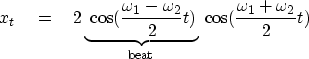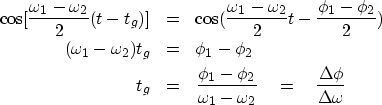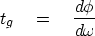Next: Group delay as a Up: PHASE DELAY AND GROUP Previous: Phase delay

## Group delay

A more interesting kind of delay is group delay," corresponding to group velocity in wave-propagation theory. Often the group delay is nothing more than the phase delay. This happens when the phase delay is independent of frequency. But when the phase delay depends on frequency, then a completely new velocity, the group velocity," appears. Curiously, the group velocity is not an average of phase velocities.

The simplest analysis of group delay begins by defining a filter input xt as the sum of two frequencies:(26)
By using a trigonometric identity,(27)
we see that the sum of two cosines looks like a cosine of the average frequency multiplied by a cosine of half the difference frequency. Since the frequencies in Figure 17 are taken close together,beat
Figure 17
Two nearby frequencies beating.

the difference frequency factor in (28) represents a slowly variable amplitude multiplying the average frequency. The slow (difference frequency) modulation of the higher (average) frequency is called beating.''

The beating phenomenon is also called interference,'' although that word is deceptive. If the two sinusoids were two wave beams crossing one another, they would simply cross without interfering. Where they are present simultaneously, they simply add.

Each of the two frequencies could be delayed a different amount by a filter, so take the output of the filter yt to be(28)
In doing this, we have assumed that neither frequency was attenuated. (The group velocity concept loses its simplicity and much of its utility in dissipative media.) Using the same trigonometric identity on (29) as we used to get (28), we find that(29)
Rewriting the beat factor in terms of a time delay tg, we now have(30) (31)
For a continuum of frequencies, the group delay is(32)Next: Group delay as a Up: PHASE DELAY AND GROUP Previous: Phase delay
Stanford Exploration Project
10/21/1998